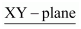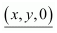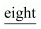• +91 9971497814
• info@interviewmaterial.com

# Chapter 12 Introduction to Three Dimensional Geometry Ex-12.1 Interview Questions Answers

### Related Subjects

Question 1 : A point is on the x-axis. What are its y-coordinates and z-coordinates?

Answer 1 : If a point is on the x-axis, then its y-coordinates and z-coordinates are zero.

Question 2 : A point is in the XZ-plane. What can you say about its y-coordinate?

Answer 2 : If a point is in the XZ plane, then its y-coordinate is zero.

Question 3 :
Name the octants in which the following points lie:
(1, 2, 3), (4, –2, 3), (4, –2, –5), (4, 2, –5), (–4, 2, –5), (–4, 2, 5), (–3, –1, 6), (2, –4, –7)

The x-coordinate, y-coordinate, and z-coordinate of point (1, 2, 3) are all positive. Therefore, this point lies in octant I.
The x-coordinate, y-coordinate, and z-coordinate of point (4, –2, 3) are positive, negative, and positive respectively. Therefore, this point lies in octant IV.
The x-coordinate, y-coordinate, and z-coordinate of point (4, –2, –5) are positive, negative, and negative respectively. Therefore, this point lies in octant VIII.
The x-coordinate, y-coordinate, and z-coordinate of point (4, 2, –5) are positive, positive, and negative respectively. Therefore, this point lies in octant V.
The x-coordinate, y-coordinate, and z-coordinate of point (–4, 2, –5) are negative, positive, and negative respectively. Therefore, this point lies in octant VI.
The x-coordinate, y-coordinate, and z-coordinate of point (–4, 2, 5) are negative, positive, and positive respectively. Therefore, this point lies in octant II.
The x-coordinate, y-coordinate, and z-coordinate of point (–3, –1, 6) are negative, negative, and positive respectively. Therefore, this point lies in octant III.
The x-coordinate, y-coordinate, and z-coordinate of point (2, –4, –7) are positive, negative, and negative respectively. Therefore, this point lies in octant VIII.

Question 4 : Fill in the blanks:
(i) The x-axis and y-axis taken together determine a plane known as ............. .
(ii) The coordinates of points in the XY-plane are of the form.................. .
(iii) Coordinate planes divide the space into..................  octants.

(i) The x-axis and y-axis taken together determine a plane known as.
(ii) The coordinates of points in the XY-plane are of the form.
(iii) Coordinate planes divide the space intooctants.

Todays Deals### Chapter 12 Introduction to Three Dimensional Geometry Ex-12.1 Contributorskrishan

Name:
Email:

# Latest News# 9000 interview questions in different categories